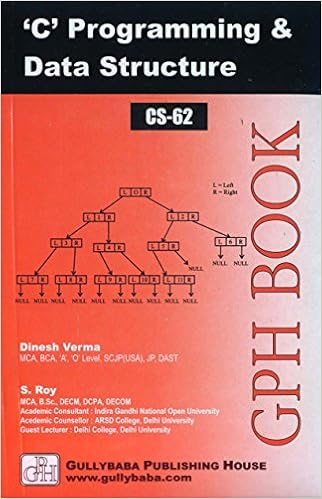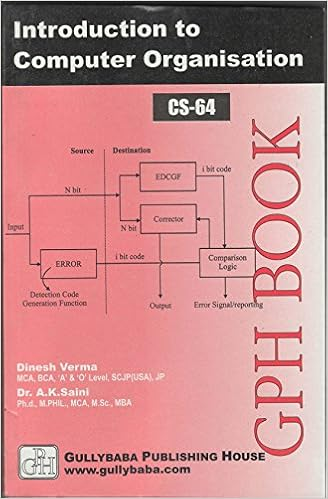IGNOU BCA MCA.comQuestion Papers of IGNOU MCA-OLD CS51

 CS51: Operation ResearchYear: 1998 TEE: December Time 2 Full Marks 75 Note: There are 6 questions in this paper. Question no. 1 is compulsory and carries 35 marks. From the remaining questions, attempt any two. Each of these carries 20 marks.Q.1(a): Define the following terms very briefly, typically within one paragraph. Pay-off matrix for a gameEOQ in the context of inventoryConstraints in an L.P. problemThe value of a gamePrior probability Q.1(b): If a transportation problem has p factories and q retail shops, what is the number of variables and what is the number of constraints?: Q.1(c): What is convex set? Illustrate with examples.: Q.2(a): List the factors that constitutes the basic elements of a queuing model. What are the assumptions made in M/M/I? What conclusions do these lead to about the average queue length?: home || Next >>